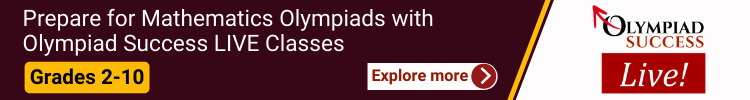IAIS Mathematics Olympiad IAIS Mathematics Olympiad Preparation Materials and Sample Papers for class 3,4,5,6,7,8,9,10

All fields are required

International Assessment for Indian Schools

# International Assessment for Indian Schools Mathematics (IAIS Mathematics)

We help students of class 1 to 10 in preparation of IAIS Mathematics exam and courses through sample question and practice papers.

About International Assessment For Indian Schools Mathematics (IAIS Mathematics)

• There are several Multiple choice questions and free response questions.
• Students need to write the answers in the boxes provided for the free response questions.
• Worth of each question is one mark.
• The total score is the number of correct answers.
• There’s no negative marking.Syllabus and Marking Scheme

Classes

No. of questions

Duration

3 to 5

40

1 Hr.

6 to 12

35

1 Hr. 20 Mins

Schedule for 2016-2017 examination

The exam will be held on 15th September, 2017.

Benefits

• IAIS delivers the unpretentious, unequalled, global assessments to you.
• It helps to identify student’s strengths and weaknesses.
• A lot of valuable experience is gained while taking these international tests.
##### Class 1

What we Offer?

• Comprehensive Test Series: 60+ worksheets + 10+ mock test papers
• Mock Test Series: 10+ mock test papers (IAIS Mathematics Mock Test Papers are based on Previous Year question papers for Class 1)

* 20+ logical reasoning worksheets complimentary for every subscriber

##### Class 2
What we Offer?

• Comprehensive Test Series: 40+ worksheets + 20+ mock test papers
• Mock Test Series: 20+ mock test papers (IAIS Mathematics Mock Test Papers are based on Previous Year question papers for Class 2)
* 20+ logical reasoning worksheets complimentary for every subscriber
##### Class 3

Number & Arithmetic, Algebra & Patterns, Measure & Units, Space & Geometry, Chance & Data.

What we Offer?

• Comprehensive Test Series: 60+ worksheets + 10+ mock test papers
• Mock Test Series: 10+ mock test papers (IAIS Mathematics Mock Test Papers are based on Previous Year question papers for Class 3)

* 20+ logical reasoning worksheets complimentary for every subscriber

##### Class 4

Number & arithmetic, Algebra & patterns, measure & units, Space & geometry, Chance & data.

What we Offer?

• Comprehensive Test Series: 60+ worksheets + 10+ mock test papers
• Mock Test Series: 10+ mock test papers (IAIS Mathematics Mock Test Papers are based on Previous Year question papers for Class 4)

* 20+ logical reasoning worksheets complimentary for every subscriber

##### Class 5

Number & arithmetic, Algebra & patterns, measure & units, Space & geometry, Chance & data.

What we Offer?

• Comprehensive Test Series: 60+ worksheets + 10+ mock test papers
• Mock Test Series: 10+ mock test papers (IAIS Mathematics Mock Test Papers are based on Previous Year question papers for Class 5)

* 20+ logical reasoning worksheets complimentary for every subscriber

##### Class 6

Number & arithmetic, Algebra & patterns, measure & units, Space & geometry, Chance & data.

What we Offer?

• Comprehensive Test Series: 60+ worksheets + 10+ mock test papers
• Mock Test Series: 10+ mock test papers (IAIS Mathematics Mock Test Papers are based on Previous Year question papers for Class 6)

* 20+ logical reasoning worksheets complimentary for every subscriber

##### Class 7

Number & arithmetic, Algebra & patterns, measure & units, Space & geometry, Chance & data.

What we Offer?

• Comprehensive Test Series: 60+ worksheets + 10+ mock test papers
• Mock Test Series: 10+ mock test papers (IAIS Mathematics Mock Test Papers are based on Previous Year question papers for Class 7)

* 20+ logical reasoning worksheets complimentary for every subscriber

##### Class 8

Number & arithmetic, Algebra & patterns, measure & units, Space & geometry, Chance & data.

What we Offer?

• Comprehensive Test Series: 60+ worksheets + 10+ mock test papers
• Mock Test Series: 10+ mock test papers (IAIS Mathematics Mock Test Papers are based on Previous Year question papers for Class 8)

* 20+ logical reasoning worksheets complimentary for every subscriber

##### Class 9

Number & arithmetic, Algebra & patterns, measure & units, Space & geometry, Chance & data.

What we Offer?

• Comprehensive Test Series: 60+ worksheets + 10+ mock test papers
• Mock Test Series: 10+ mock test papers (IAIS Mathematics Mock Test Papers are based on Previous Year question papers for Class 9)

* 20+ logical reasoning worksheets complimentary for every subscriber

##### Class 10

Number & arithmetic, Algebra & patterns, measure & units, Space & geometry, Chance & data.

What we Offer?

• Comprehensive Test Series: 60+ worksheets + 10+ mock test papers
• Mock Test Series: 10+ mock test papers (IAIS Mathematics Mock Test Papers are based on Previous Year question papers for Class 10)

* 20+ logical reasoning worksheets complimentary for every subscriber